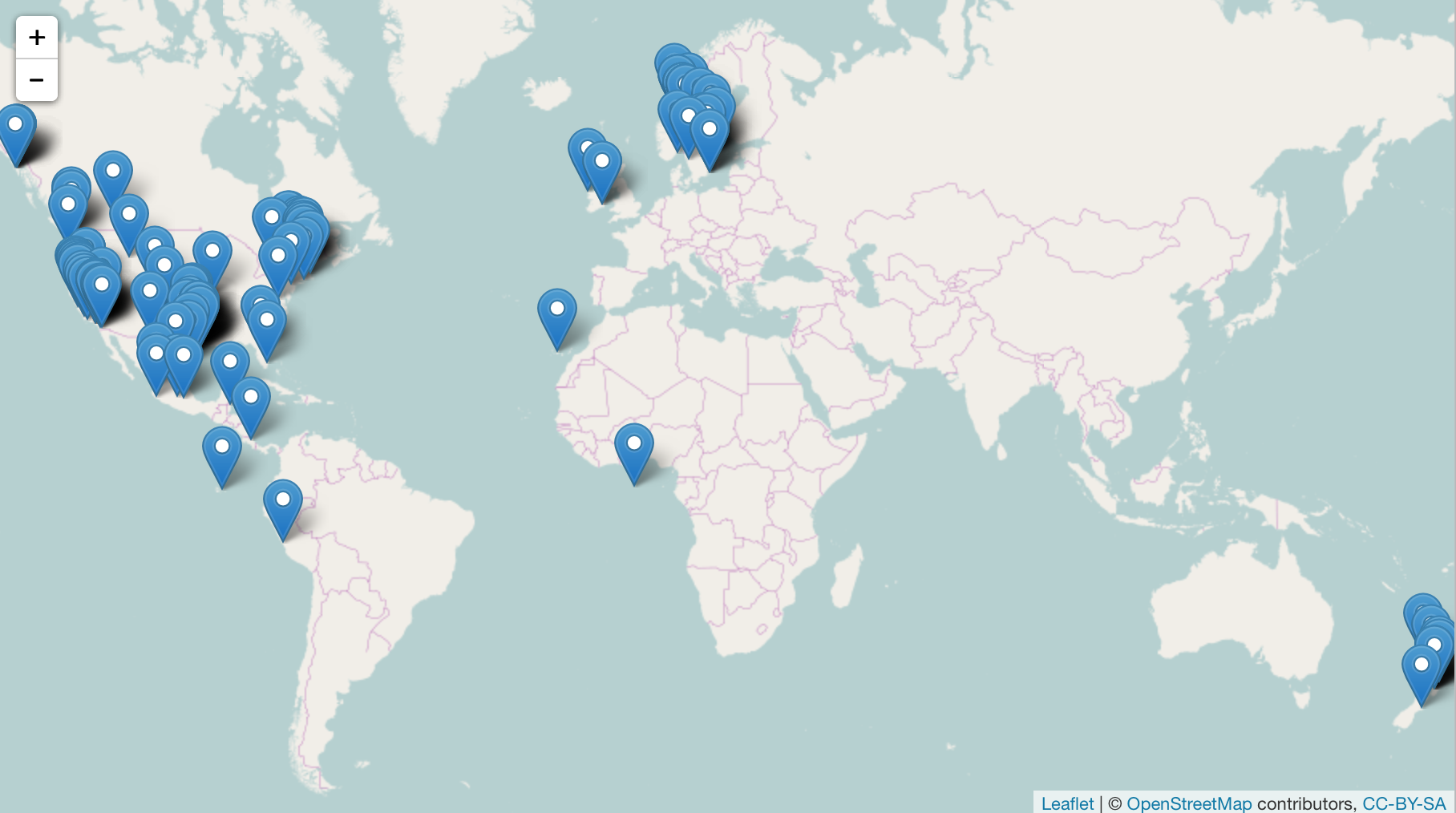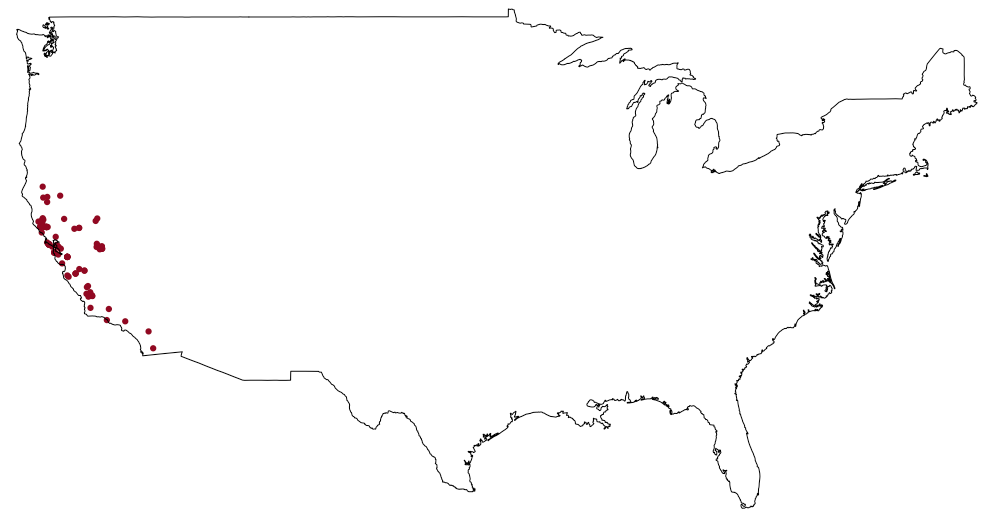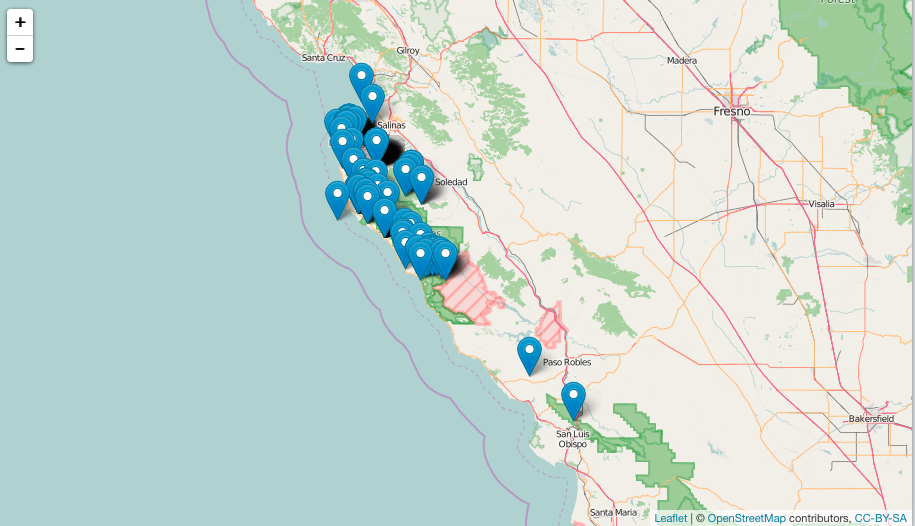Helper for making maps of species occurrence data, including for:

This package has utilities for making maps with:

• base R
• ggplot2
• ggplot2 with map layers - via `ggmap` pkg
• Leaflet - via `leaflet` pkg
• GitHub Gists - via `gistr` package

## Installation

Install `mapr`

``install.packages("mapr", dependencies = TRUE)``

Or the development version from GitHub

``devtools::install_github("ropensci/mapr")``
``````library("mapr")
library("spocc")``````

## Make maps

### Leaflet

``````spp <- c('Danaus plexippus', 'Accipiter striatus', 'Pinus contorta')
dat <- occ(query = spp, from = 'gbif', has_coords = TRUE, limit = 50)
map_leaflet(dat)``````### Github gist

``````dat <- fixnames(dat)
map_gist(dat, color = c("#976AAE","#6B944D","#BD5945"))``````### ggplot2 family maps

#### ggmaps

``````x <- occ(query = 'Lynx rufus californicus', from = 'gbif', limit = 100)
map_ggmap(x)``````#### ggplot

``map_ggplot(x, "usa")``### Base R plots

``map_plot(dat, size = 1, pch = 10)``### via dismo

if that’s your jam, though you might find `rgbif` easier

``````library("dismo")
g <- gbif('Batrachoseps', 'luciae', geo = TRUE, end = 300)
map_leaflet(g, lon = "lon", lat = "lat", name = "scientificName")``````## Meta

• Please report any issues or bugs.
• Get citation information for `mapr` in R doing `citation(package = 'mapr')`